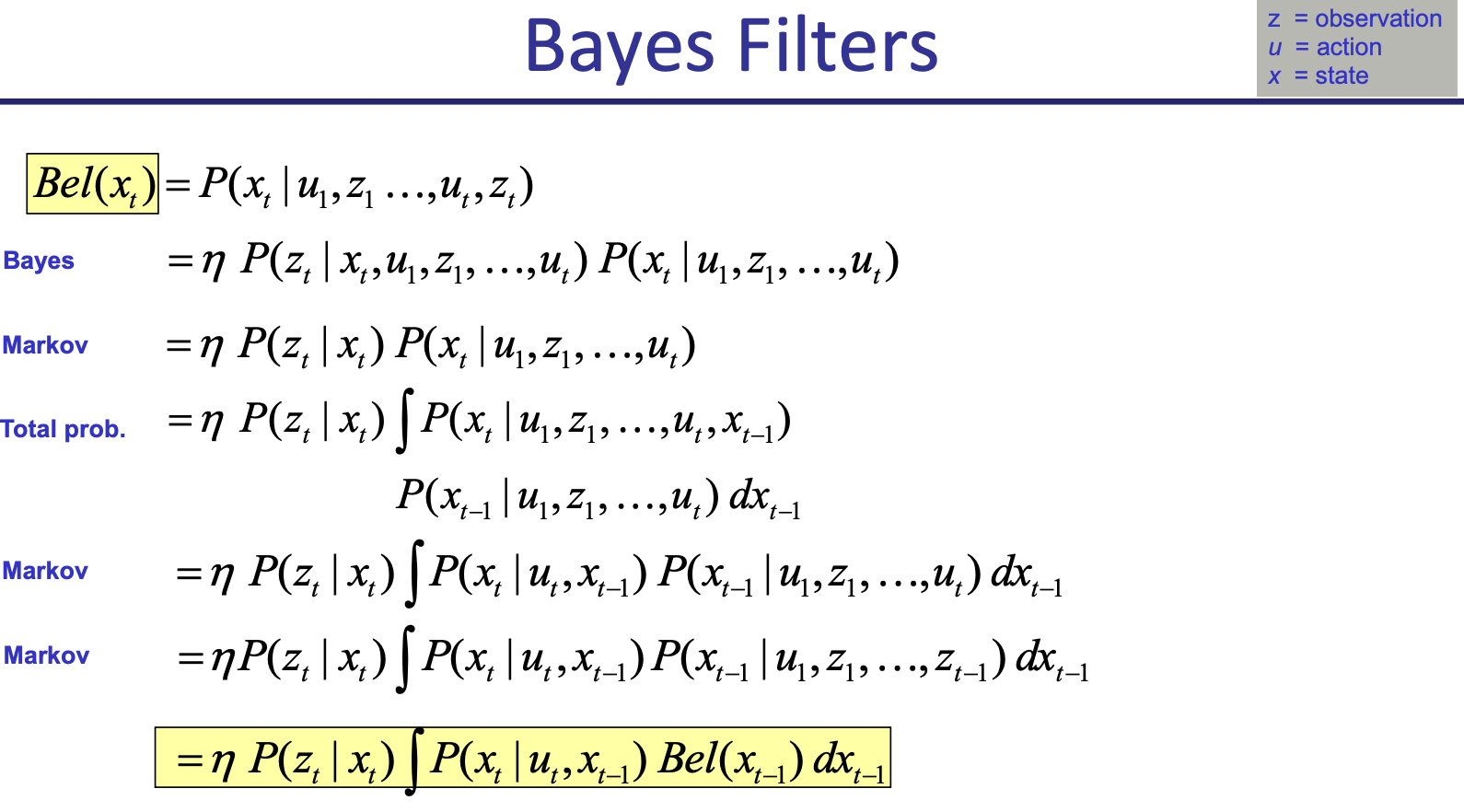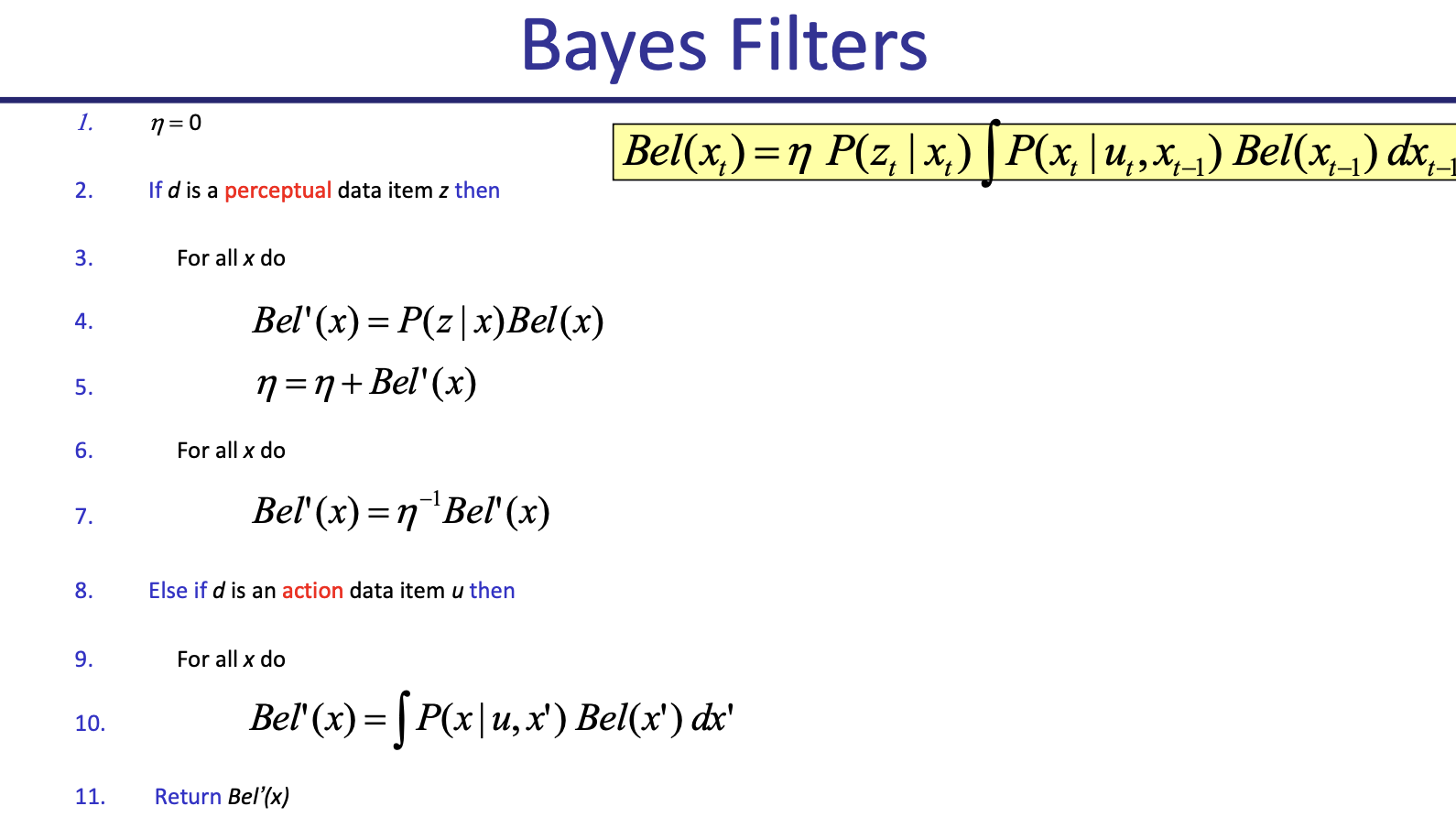State Estimation

# Bayes Filter §

A Bayes filter is a recursive algorithm that uses Bayes Rule to perform State Estimation.

Given:

• Stream of observations and action data
• Sensor model
• Action model
• Prior probability of the system state

We want an estimate of the state of a dynamical system, essentially , so we use Bayes Rule

We do doing using by updating the posterior of the state, which we call belief:

Bayes Filter allow us to recursively update our belief state using the equation where

• is the normalization constant, to make sure everything adds up to 1
• is the observation Likelihood sensor model
• Transition model, motion model (simulate noisy dynamics of particles based on control input)

Important

Bayes rule make use of the Markov Assumption.

Derivation from CS287We can then come up with an algorithm to do this: